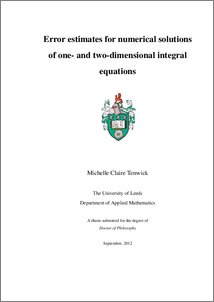# Error estimates for numerical solutions of one- and two-dimensional integral equations

Tenwick, Michelle Claire (2012) Error estimates for numerical solutions of one- and two-dimensional integral equations. PhD thesis, University of Leeds.Preview
Text
Michelle_Tenwick_Thesis.pdf